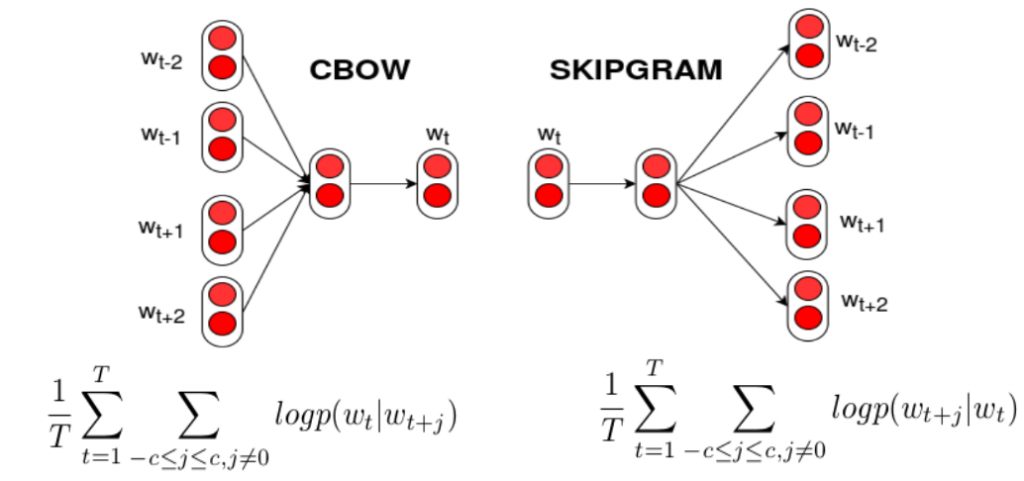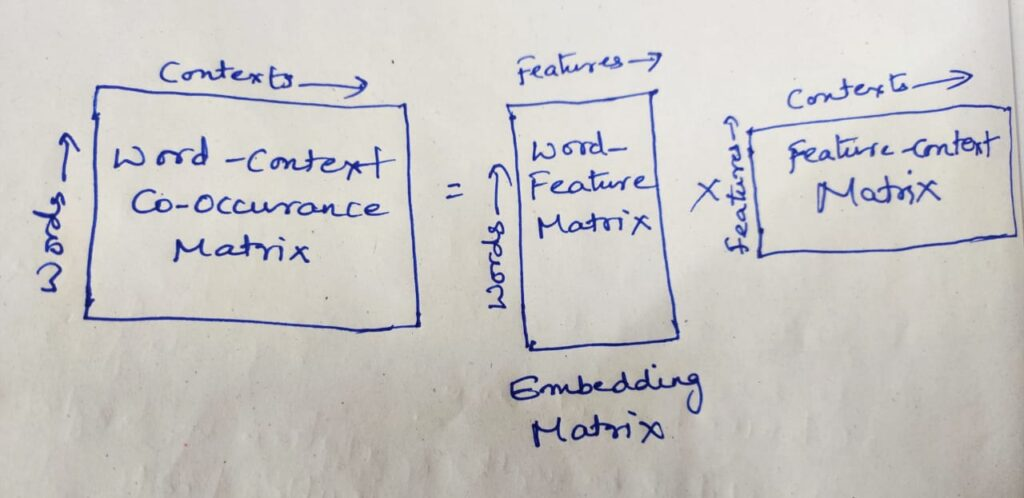# What is the difference between word2Vec and Glove ?

Both word2vec and glove enable us to represent a word in the form of a vector (often called embedding). They are the two most popular algorithms for word embeddings that bring out the semantic similarity of words that captures different facets of the meaning of a word.

They are used in many NLP applications such as sentiment analysis, document clustering, question answering, paraphrase detection and so on.  Pretrained models for both these embeddings are readily available and they are easy to incorporate into python code.

What is the difference between the two models?: Word2vec embeddings are based on training a shallow feedforward neural network while glove embeddings are learnt based on matrix factorization techniques.

However, to get a better understanding let us look at the similarity and difference in properties for both these models, how they are trained and used.

### Properties of both word2vec and glove:

1. The relationship between words is derived by cosine distance between words. Such word vectors are good at answering analogy questions. For instance, in the picture below, we see that the distance between king and queen is about the same as that between man and woman2. Semantically similar words are close together. Consider two words such as learn and study. These words are closer to each other in cosine distance compared to ‘learn‘ and ‘eat‘.
3. We can also use arithmetic on these embeddings to derive meaning. For instance in the example below, we see that “Berlin-Germany+France=Paris”.

4.  We can obtain phrasal Embeddings by adding up word embeddings: Since we can perform arithmetic operations on these vectors in a way that can preserve their semantics, one can find an embedding for a phrase by adding up embedding for individual words.

As a result, in several applications, even though two sentances or documents do not have words in common, their semantic similarity can be captured by comparing the cosine similarity in the phrasal embeddings obtained by adding up indivudual word embeddings.

### Differences in the properties of word2vec and glove:

The two models differ in the way they are trained, and hence lead to word vectors with subtly different properties. Glove model is based on leveraging global word to word co-occurance counts leveraging the entire corpus. Word2vec on the other hand leverages co-occurance within local context (neighbouring words).

In practice, however, both these models give similar results for many tasks. ​_Factors such as the dataset on which these models are trained, length of the vectors and so on seem to have a bigger impact than the models themselves. For instance, if I am using these models to derive  the features for a medical application, I can significantly improve performance by training on dataset from the medical domain.

### How are the word2vec and glove models trained:

How is the word2vec model trained?

Word2Vec is a feed forward neural network based model to find word embeddings. There are two models that are commonly used to train these embeddings: The skip-gram and the CBOW model.

The Skip-gram model takes the input as each word in the corpus, sends them to a hidden layer (embedding layer) and from there it predicts the context words. Once trained, the embedding for a particular word is obtained by feeding the word as input and taking the hidden layer value as the final embedding vector.

The CBOW (Continuous Bag of Words) model takes the input the context words for the given word, sends them to a hidden layer (embedding layer) and from there it predicts the original word. Once again, after training, the embedding for a particular word is obtained by feeding the word as input and taking the hidden layer value as the final embedding vector.

The following figure pictorially shows these models.How is the Glove model trained?

Glove is based on matrix factorization techniques on the word-context matrix. It first constructs a large matrix of (words x context) co-occurrence information, i.e. for each “word” (the rows), you count how frequently we see this word in some “context” (the columns) in a large corpus.  The number of “contexts” is of course large, since it is essentially combinatorial in size.

So then we factorize this matrix to yield a lower-dimensional (word x features) matrix, where each row now yields a vector representation for the corresponding word. In general, this is done by minimizing a “reconstruction loss”. This loss tries to find the lower-dimensional representations which can explain most of the variance in the high-dimensional data.How can you use word2vec and glove models in your code?

The following pieces of code show how one can use word2vec and glove in their project. A more detailed coding example on word embeddings and various ways of representing sentences is given in this hands-on tutorial with source code.

How can you use the word2vec pretrained model in your code?

```from gensim.models import Word2Vec

sample_word2vec_embedding=v2w_model['computer'];```

How can you use the Glove pretrained model in your code?

```import gensim.downloader as api